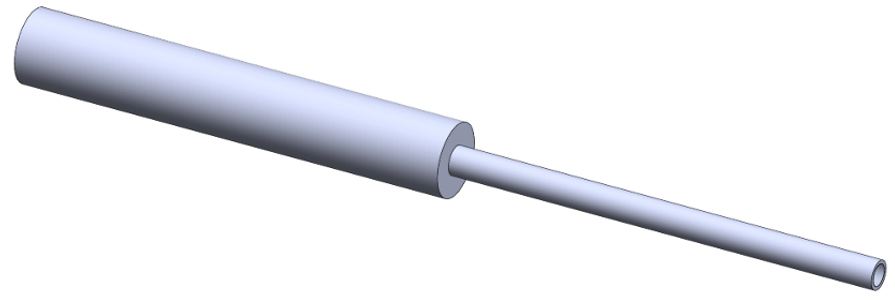LearnChemE

#### Dimensional Analysis, Pi Terms, and Dimensionless Numbers: Example Problems

Try to solve these problems before watching the solutions in the screencasts.

##### Example Problem 1

A viscous fluid flows through this contraction. You need to estimate the pressure drop, ΔP, that is caused by the contraction. Bernoulli’s equation cannot be used because the fluid is quite viscous, and the N-S equations cannot be simplified enough to integrate. Thus, you’ll need experimental data to find ΔP. Determine all dimensionless groups (Π-terms) needed to analyze this situation with experiments. The variables that will likely affect ΔP are density, ρ, dynamic viscosity, μ, the diameter of the large pipe, D1, the diameter of the narrow pipe, D2, and the average speed of the fluid through the large pipe, V1.##### Example Problem 2

A small raindrop lands in a puddle, causing waves to propagate outward from the point where it lands. You wish to investigate variables that affect the speed at which the waves propagate outward. You need results that are applicable to all sorts of Newtonian fluids, not just water. You make the assumption that the speed of wave propagation, V, depends on four variables: the density of the liquid, ρ, the distance between the crests of two waves, λ, the wave height, h, and the surface tension of the liquid, σ. Form dimensionless groups ( terms) that you can use to analyze your experiments.